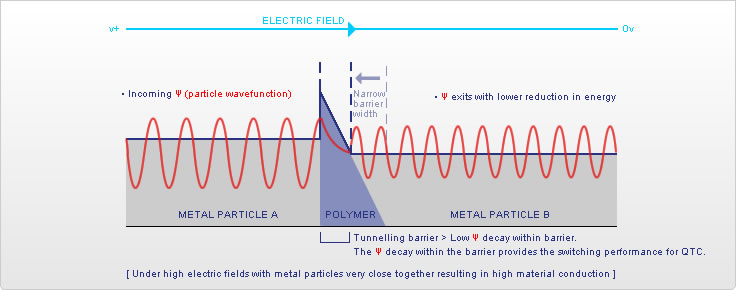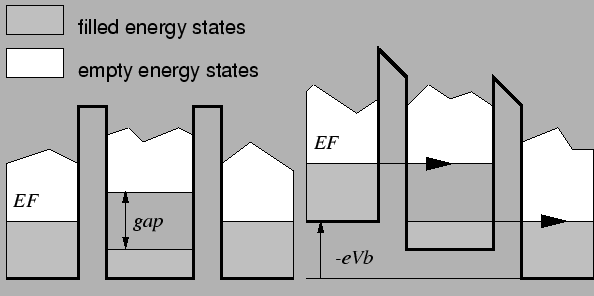# This note has been used to help create the Quantum Tunneling wikiTransmission and Reflection

My past lectures have been largely theoretical and mathematically intensive. That was to introduce the foundations or "language" in which QM works. Now it is time to look into actual physical phenomena, which will lead us into the wonderful applications that have helped us forge the modern world.

If you look into my note Basic Quantum Objects, you will notice that every object is one-dimensional and perhaps artificial. However, each case is in fact a model for materials, and electronic devices found in everyday life. For now let us investigate the rectangular barrier.Let us separate the picture into three regions:

1. Region 1 $(-\infty < x<0)$: $E > V$

2. Region 2 $(0 \le x \le L)$: $E \le V$

3. Region 3 $(L < x< \infty)$: $E > V$

In Region 1, the potential is zero. A moving wave thus has energy is greater than the potential. This is also true in Region 3. However, in Region 2, the energy of the wave is either less than or equal to the potential. Thus from the Schrödinger equation, we find two differential equations:

1. Region 1 and Region 3 $\frac{{d}^{2}\psi}{d{x}^{2}}={k}^{2}\psi, \quad k=\sqrt{\frac{-2mE}{{\hbar}^{2}}}$

2. Region 2 $\frac{{d}^{2}\psi}{d{x}^{2}}={\kappa}^{2}\psi, \quad \kappa=\sqrt{\frac{2m(V-E)}{{\hbar}^{2}}}$

Hence we find that the wavefunctions take the form:

$\psi (x)=\begin{cases} { Ae }^{ ikx }+{ Be }^{ -ikx } \quad, \text{Region 1} \\ { Ce }^{ \kappa x }+{ De }^{ -\kappa x } \quad , \text{Region 2} \\ { Fe }^{ ikx } \quad \quad \quad \quad , \text{Region 3} \end{cases}$

If you were wondering where ${Ge}^{-ikx}$ went in the solution of the above differential equations, you should know what the exponents mean. Plane-waves that travel to the right are of the form ${e}^{ikx}$ and plane-waves that travel to the left are of the form ${e}^{-ikx}$. In quantum tunnelling, there are waves that get reflected and transmitted, so in Region 1 we find waves travelling to the left (heading towards the barrier and transmitted) and waves travelling to the left (reflected by the barrier); whereas, the wave travels solely to the right in Region 3.

The continuity of wavefunctions and its derivative wavefunctions allows us to solve this problem. From the continuity condition we get:

1. $A+B=C+D$

2. ${Ce}^{\kappa L}+{De}^{-\kappa L}= {Fe}^{ikL}$

3. $Aik-Bik=C\kappa-D\kappa$

4. ${C\kappa e}^{\kappa L}-{D\kappa e}^{-\kappa L}= {Fike}^{ikL}$

Therefore by making combinations of the above equations we find (try this yourself)

1. $2A=\left(1+\frac{\kappa}{ik}\right)C+\left(1-\frac{\kappa}{ik}\right)D$

2. $2C{e}^{\kappa L}=\left(1+\frac{ik}{\kappa}\right)F{e}^{ikL}$

3. $2D{e}^{-\kappa L}=\left(1-\frac{ik}{\kappa}\right)F{e}^{ikL}$

Combining these three equations yields

$2A=\left(1+\frac{\kappa}{ik}\right)\left(1+\frac{ik}{\kappa}\right)\frac{F{e}^{ikL}{e}^{-\kappa L}}{2}+\left(1-\frac{\kappa}{ik}\right)\left(1-\frac{ik}{\kappa}\right)\frac{F{e}^{ikL}{e}^{\kappa L}}{2}$

which can be simplified as

$\frac{A{e}^{-ikL}}{F}=\cosh{(\kappa L)}+i\left(\frac{{\kappa}^{2}-{k}^{2}}{2k\kappa}\right)\sinh{(\kappa L)}$

Now the probability of a wave to tunnel through the barrier is equal to the probability of the wavefunction in Region 3 divided by the probability of the wavefunction in Region 1. Thus the probability of transmission is quantified by

$T=\frac{{F}^{*}F}{{A}^{*}A}={\left[{{\text{cosh}}^{2}(\kappa L)+\left(\frac{{\kappa}^{2}-{k}^{2}}{2k\kappa}\right)}^{2}{\text{sinh}}^{2}(\kappa L)\right]}^{-1}$

(remember probabilities are defined by the complex conjugate product).

Since ${\text{cosh}}^{2}(x)-{\text{sinh}}^{2}(x)=1$,

$T={\left[1+\left(\frac{{k}^{2}+{\kappa}^{2}}{2k\kappa}\right){\text{sinh}}^{2}(\kappa L)\right]}^{-1}.$

If we let $\beta=\left(\frac{{k}^{2}+{\kappa}^{2}}{2k\kappa}\right)$, we find

$T=\frac{1}{1+{\beta}^{2}{\text{sinh}}^{2}(\kappa L)}.$

Naturally, the probability of reflection is $1-T$; hence,

$R=\frac{{\beta}^{2}{\text{sinh}}^{2}(\kappa L)}{1+{\beta}^{2}{\text{sinh}}^{2}(\kappa L)}.$

In our macroscopic experience, objects colliding against a wall will be deflected. This is analogous to the reflection probability being 100% and transmission probability being 0%. The above example shows that it is possible for matter waves to "go through walls" with some probability, given that a matter wave has sufficient energy or the barrier being sufficiently narrow (small L).

Check out this video

Applications of Quantum Tunnelling

Quantum tunnelling is responsible for many physical phenomena that baffled scientists in the early 20th century. The first would be radioactivity. The most common type of radioactive decay is caused by an electron being captured into atomic nuclei. Contrary to the Bohr model of the atom, electrons exist not in shells but in "clouds" of probability density. Because quantum tunnelling is common at the atomic and subatomic levels this electron capture is nothing strange. Ever since, quantum tunnelling has played a vital role in our capability of manipulating electrons.

Other applications of quantum tunnelling include:

Scanning Tunnelling MicroscopeP-N junctionTunnel junctionVisit my set Lectures on Quantum Mechanics for more notes.

ProblemsNote by Steven Zheng
6 years, 4 months ago

This discussion board is a place to discuss our Daily Challenges and the math and science related to those challenges. Explanations are more than just a solution — they should explain the steps and thinking strategies that you used to obtain the solution. Comments should further the discussion of math and science.

When posting on Brilliant:

• Use the emojis to react to an explanation, whether you're congratulating a job well done , or just really confused .
• Ask specific questions about the challenge or the steps in somebody's explanation. Well-posed questions can add a lot to the discussion, but posting "I don't understand!" doesn't help anyone.
• Try to contribute something new to the discussion, whether it is an extension, generalization or other idea related to the challenge.
• Stay on topic — we're all here to learn more about math and science, not to hear about your favorite get-rich-quick scheme or current world events.

MarkdownAppears as
*italics* or _italics_ italics
**bold** or __bold__ bold
- bulleted- list
• bulleted
• list
1. numbered2. list
1. numbered
2. list
Note: you must add a full line of space before and after lists for them to show up correctly
paragraph 1paragraph 2

paragraph 1

paragraph 2

[example link](https://brilliant.org)example link
> This is a quote
This is a quote
    # I indented these lines
# 4 spaces, and now they show
# up as a code block.

print "hello world"
# I indented these lines
# 4 spaces, and now they show
# up as a code block.

print "hello world"
MathAppears as
Remember to wrap math in $$ ... $$ or $ ... $ to ensure proper formatting.
2 \times 3 $2 \times 3$
2^{34} $2^{34}$
a_{i-1} $a_{i-1}$
\frac{2}{3} $\frac{2}{3}$
\sqrt{2} $\sqrt{2}$
\sum_{i=1}^3 $\sum_{i=1}^3$
\sin \theta $\sin \theta$
\boxed{123} $\boxed{123}$

## Comments

Sort by:

Top Newest

Hi sir. Sorry but most of your notes on QM are a total overhead bouncer for me . But I am very much interested in QM, can you please suggest some sources . I know that even I can search on the Internet and find it out myself but an Expert's advice is always the best !!

Thanks for the same .

- 6 years, 4 months ago

Log in to reply

Start with "Introduction to quantum mechanics" by Griffiths. Make sure you attempt all the difficult problems in that. After that there are many books. Some standard ones are by, "Sakurai", "Shankar", "Gottfried", "Dirac"... it's a long list (really!) You can also do Feynman Lectures (Volume 3) alongside. This is not very mathematical, but it's a good choice for a beginner. All the best!

- 6 years, 3 months ago

Log in to reply

Griffiths is my main source. It's a good one to learn from because he goes into a lot of applications. I was surprised that he barely talked about the conservation of probability, which to me is quite strange.

- 6 years, 3 months ago

Log in to reply

Thanks a lot sir :)

- 6 years, 3 months ago

Log in to reply

Thanks a lot sir :)

- 6 years, 3 months ago

Log in to reply

×

Problem Loading...

Note Loading...

Set Loading...